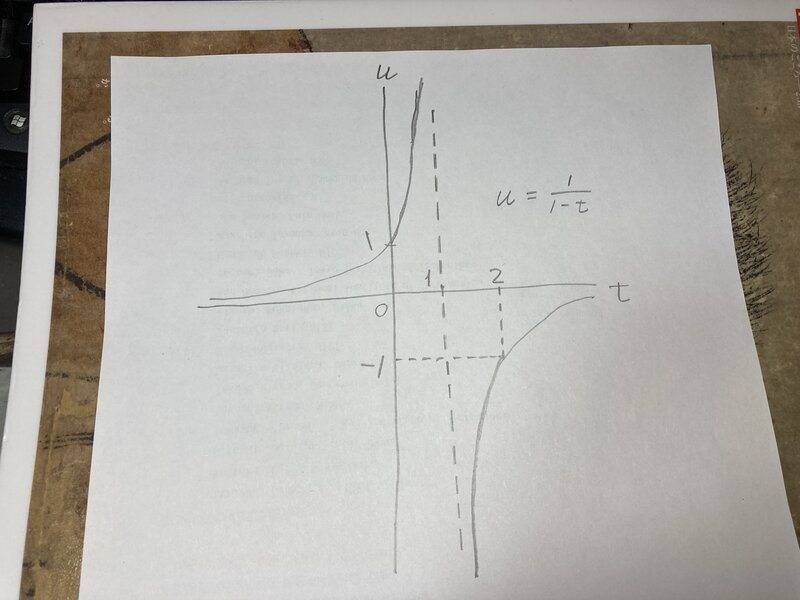# Finite difference scheme for u'=u^2, u0=1, 0<t<2

• I
feynman1
u'(t)=u(t)^2, u(0)=1, 0<t<2
What finite difference scheme can overcome the difficulty when t->1+ and help the solution jump to the negative branch?

Last edited:

Gold Member
$$\frac{du}{u^2}=dt$$
$$\frac{1}{u}+t=const.=1$$
Negative branch ?

feynman1
$$\frac{du}{u^2}=dt$$
$$\frac{1}{u}+t=const.=1$$
Negative branch ?
when t>1, u<0

Gold Member
Yes.
$$u=\frac{1}{1-t}$$
What's wrong with it ?

feynman1
$$u=\frac{1}{1-t}$$
What's wrong with it ?
Starting from t=0, when t->1+, finite differences fail.

Gold Member
Yes, but why do you estimate it fails ?Last edited:
feynman1
just try numerics yourself and you'll see.

Gold Member
The solution says
$$|u|>1$$
, or
$$|1/u|<1$$
if you do not like divergence to ##\pm## infinity. {1/u} (0) = 0.

Homework Helper
2022 Award
Starting from t=0, when t->1+, finite differences fail.

The domain of the initial value problem at $t = 0$ does not extend past $t \to 1^{-}$.

An antiderivative of $1/(t-1)^2$ looks like $$f: t \mapsto \begin{cases} 1/(1- t) + c_1 & t < 1 \\ 1/(1 - t) + c_2 & t > 1.\end{cases}$$ The arbitrary constants $c_1$ and $c_2$ don't have to be equal, and the constraint that $u(0) = 1$ only fixes $c_1 = 0$.

If you want to look at $t > 1$ then you must start from an initial $t_0 > 1$ - for example $u = -1$ when $t = 2$.

•feynman1